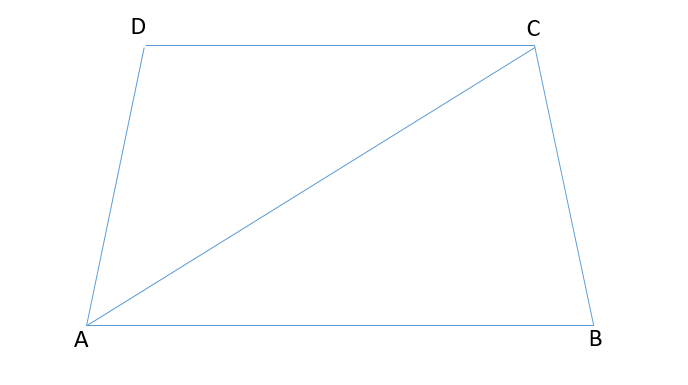Home/Class 8/Maths/

The sum of the angles of a quadrilateral is （ ）.
A. $$180^{\circ }$$
B. $$270^{\circ }$$
C. $$360^{\circ }$$
D. $$300^{\circ }$$Speed
00:00
02:49## QuestionMathsClass 8

The sum of the angles of a quadrilateral is （ ）.
A. $$180^{\circ }$$
B. $$270^{\circ }$$
C. $$360^{\circ }$$
D. $$300^{\circ }$$

C
4.64.6## Solution$$\text{Sum of angles of quadrilateral ABCD= Sum of angles of triangle ABC + Sum of angles of triangle ADC}$$.
$$\text{Sum of angles of quadrilateral ABCD} =180\degree +180\degree$$.
$$\text{Sum of angles of quadrilateral ABCD} =360\degree$$.
Hence the correct option is C.